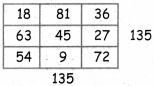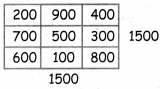Students can download 4th Maths Term 1 Chapter 3 Patterns InText Questions and Answers, Notes, Samacheer Kalvi 4th Maths Guide Pdf helps you to revise the complete Tamilnadu State Board New Syllabus, helps students complete homework assignments and to score high marks in board exams.

## Tamilnadu Samacheer Kalvi 4th Maths Solutions Term 1 Chapter 3 Patterns InText Questions

Activity (Text Book Page No. 40)Activity (Text Book Page No. 41)

Identify the patterns in multiplication and division (multiples of 6).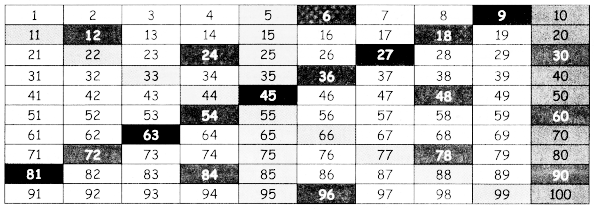Activity (Text Book Page No. 42)

Make paterns based on the multiples of 9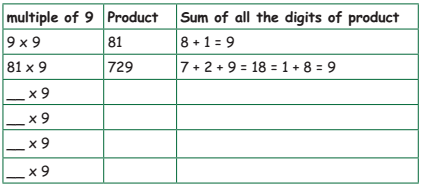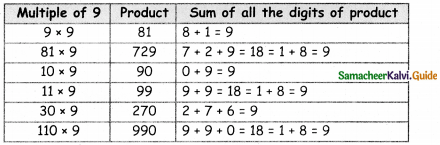Activity (Text Book Page No. 44)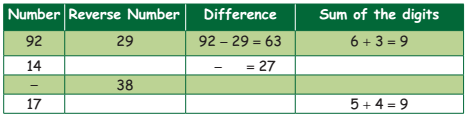Activity (Text Book Page No. 46)

Question 1.
× 200
3 → _____
2 → _____
4 → _____
5 → _____
3 → 600
2 → 400
4 → 800
5 → 1000Question 2.
× 3
60 → _____
200 → _____
30 → _____
500 → _____
60 → 180
200 → 600
30 → 90
500 → 1500

Question 3.
× 10
7 → _____
60 → _____
6 → _____
100 → _____
7 → 70
60 → 600
6 → 60
100 → 1000Question 4.
× 9
20 → _____
400 → _____
30 → _____
500 → _____
20 → 180
400 → 3600
30 → 270
500 → 4500

Activity 2 (Text Book Page No. 47)
Complete the following.
a. 54 ÷ 9 = 6
b. 540 ÷ 9 = 60
c. 5400 + 9 = ______
d. ______ ÷ 9 = 6000
c. 5400 + 9 = ______
5400 + 9 = 600Try This (Text Book Page No. 48)

Create magic squares by using,
1. Multiples of nine
2. Multiples of hundred